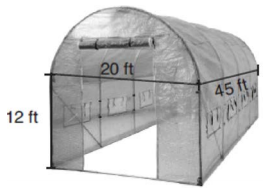mycollegehive
The greenhouse pictured below can be modeled as a rectangula...
JMAP math geometry0The greenhouse pictured below can be modeled as a rectangular prism with a half-cylinder on top. The rectangular prism is 20 feet wide, 12 feet high, and 45 feet long. The half-cylinder has a diameter of 20 feet.To the nearest cubic foot, what is the volume of the greenhouse?

1) 17,869

3) 39,074

2) 24,937

4) 67,349

657 viewsShareFollowUniversity of Benin Nigeria
23 June 2020University of Lagos Nigeria
09 December 20200Since the greenhouse comprises of a rectangular prism and half a cylinder, the volume of the railing = volume of rectangular prism + half the volume of a cylinder.

Volume of rectangular prism = L x H x W.

Where H is the height of the rectangular section of the greenhouse, H is the height of the rectangular section of the greenhouse and W is the width of the rectangular section of the greenhouse.

Thus, volume of the rectangular section of the greenhouse = 45 x 20 x 12

= 10,800

Volume of cylinder = πr2h. Where r is the radius and h is the height.

radius = diameter/2 = 20/2 = 10

volume of the cylindrical section = π x (10)2 x 45

= 4500π

Thus, half the volume = 4500π/2

= 2250π

Therefore, the total volume = 10800 + 2250π

= 17,869

Ans 1Share

### Groups

How to insert math formulas/equations### Related Tags

JMAP

12 followers

143 questionsmath

36 followers

1262 questionsgeometry

13 followers

99 questions